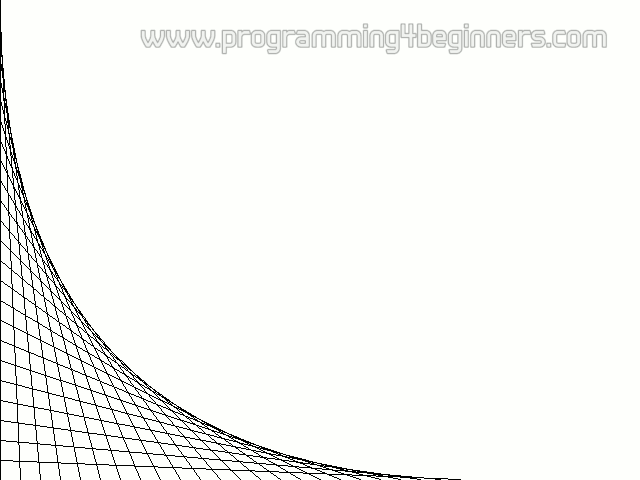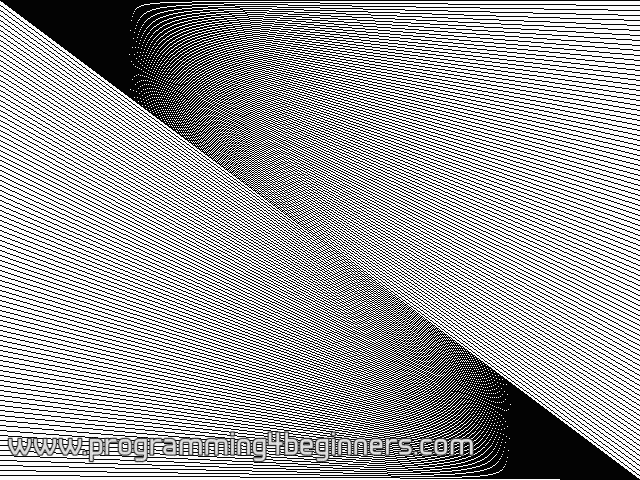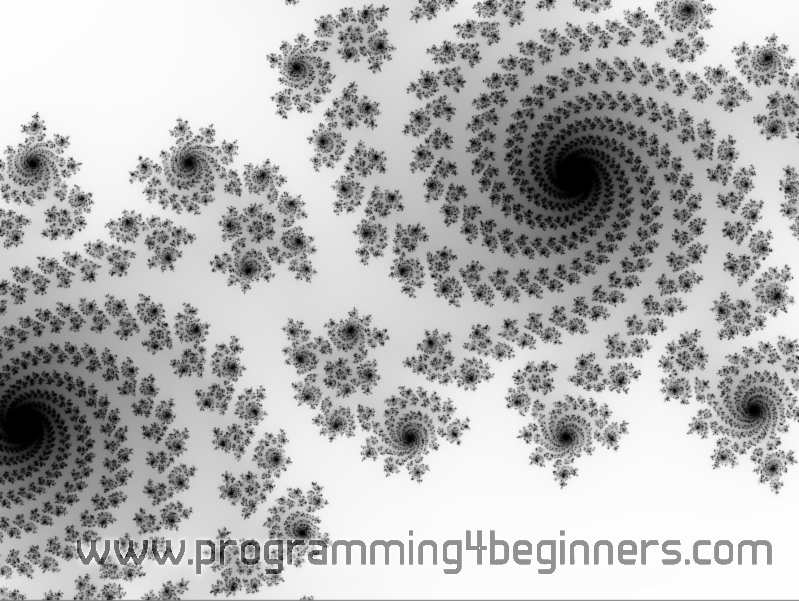## Computing a Picture

### More Drawings

The variable `i` in this `for` loop will increase by 20 in each iteration. In the first iteration, the variable `i` will have the value 0, then 20 in second iteration, then 40, 60 and so on, while the value of `i` is less than 600.

We can get some interesting effects by just drawing many lines with help of `for` loops. For example:

```    clear_to_color(surface, makecol(255,255,255));
for (int i=0;i<600;i+=20)
line(surface,0,i,i,600,makecol(0,0,0));
```This piece of code just needs to replace the part of the code that draws a human in the previous program. As a result, you should get the following picture:

Here is another interesting example:

```    clear_to_color(surface, makecol(255,255,255));
for (int i=0;i<600;i+=4)
line(surface,0,0,800,i,makecol(0,0,0));
for (int i=0;i<600;i+=4)
line(surface,800,600,0,i,makecol(0,0,0));
```The strange patterns that appear, known as moiré, are produced by the effect called aliasing.

The final demonstration program will create the entire image pixel by pixel. It will produce an image of something called a Julia set. To understand how such a simple program produces such a complex image requires some knowledge of mathematics, and we will be skipping the explanation. Instead, we will just leave you to marvel at the strange shapes called fractals that this program produces:

```#include <allegro.h>

int main()
{
if (allegro_init()!=0) return 1;
set_color_depth(32);
if (set_gfx_mode(GFX_AUTODETECT_WINDOWED, 800, 600, 0, 0)!=0)
return 2;
BITMAP* surface = create_bitmap(SCREEN_W, SCREEN_H);
install_keyboard();
for (int y=0;y<SCREEN_H;y++)
for (int x=0;x<SCREEN_W;x++) {
double re = 1.0*x/SCREEN_H - 0.5;
double im = 1.0*y/SCREEN_H - 0.5;
int n = 255;
while (n>0 && re*re+im*im<4) {
double reSq = re*re - im*im;
double imSq = 2*re*im;
re = reSq -0.629;
im = imSq +0.4;
n--;
}
putpixel(surface,x,y,makecol(n,n,n));
}
blit(surface, screen, 0,0, 0,0, SCREEN_W,SCREEN_H);
while (!keypressed())
rest(20);

return 0;
}
END_OF_MAIN()
```You can get some other interesting shapes by changing the pair of numbers (-0.629, +0.4) in the given program to some other values. We will give you a few recommendations:

• (–0.37, +0.667),
• (+0.331, +0.043),
• (–0.22, –0.72),
• (–0.758, –0.05937),
• (+0.14, +0.6),
• ( 0.278, –0.0078)